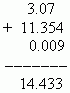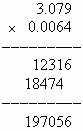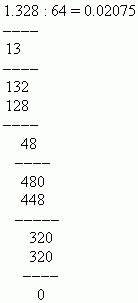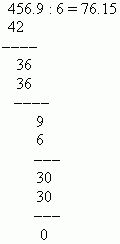# Operations with decimal fractions

Addition and subtraction of decimals. Multiplication of decimals.
Division of decimals. Division of decimal fraction by integer.
Division of decimal fraction by another one.

Addition and subtraction of decimals. These operations are executed as well as an addition and a subtraction of whole numbers. It is only necessary to write the corresponding decimal places one under another.

E x a m p l e .Multiplication of decimals. At first stage let’s multiply the fractions as integers, not taking a decimal point into consideration. After this we use the following rule: a number of decimal places in a product is equal to a sum of numbers of decimal places in all factors. Note : before putting the decimal point in the product it is prohibited  to reject zeros in the end of it !

E x a m p l e .A sum of numbers of decimal places in factors is equal: 3 + 4 = 7. A sum of digits in the product is 6. Therefore, it is necessary to add one zero to the left: 0197056  and to put before this a decimal point:  0.0197056.

Division of decimals.

Division of decimal fraction by integer.

If a dividend is less than a divisor , write zero in an integer part of a quotient and put after it a decimal point. Then, not taking the decimal point of dividend into consideration, join to its integer part the next digit of fractional part and compare again the received integer part of a dividend with a divisor. If a new number is again less than a divisor, put one more zero after a decimal point in a quotient and join to an integer part of a dividend the next digit of its fractional part. Thus, repeat this  process till the received dividend would be not more than a divisor. After this one can fulfill the division as for integers. If a dividend is more than a divisor or equal to it , divide at first its integer part, write a result of the division in the quotient and put a decimal point. After this one can continue the division as for integers.

E x a m p l e . Divide 1.328 by 64.

S o l u t i o n :Division of decimal fraction by another one.

At first transfer decimal points in a dividend and a divisor by the number of decimal places of divisor, i.e. make the divisor an integer. Now divide as well as in the previous case.

E x a m p l e . Divide 0.04569 by 0.0006.

S o l u t i o n.  Transfer the decimal points to 4 places to the right and divide 456.9 by 6: